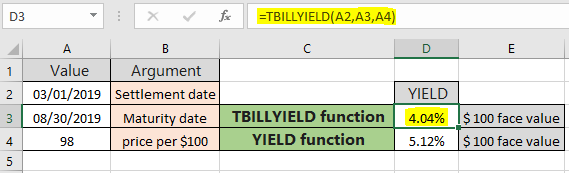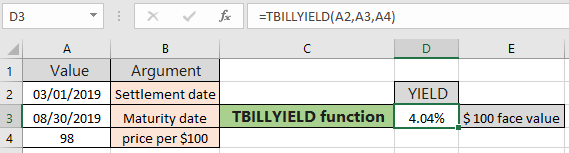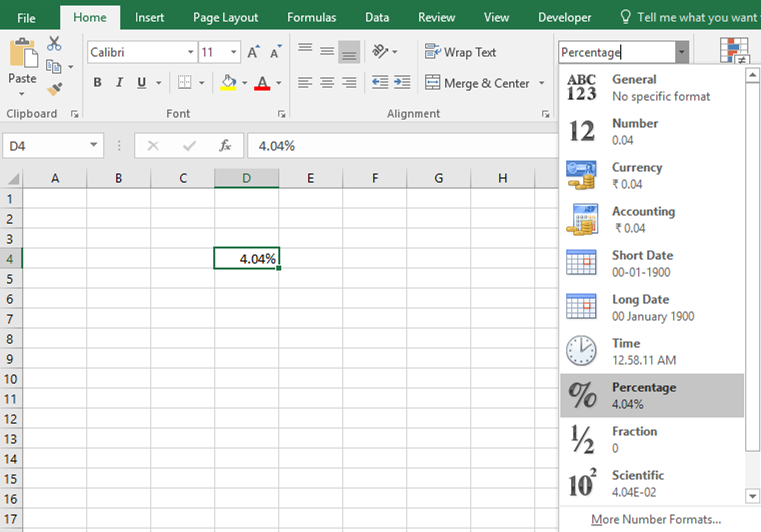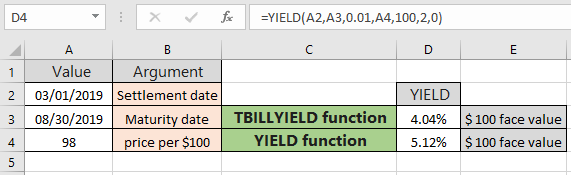# How to use the TBILLYIELD function in ExcelIn this article, we will learn How to use the TBILLYIELD function in Excel.

What is Treasury Bills (T-bill)? And how do you calculate yield on treasury bills

When the government is going to the financial market to raise money, it can do it by issuing two types of debt methods, one is treasury bills and other is government bonds. The difference between the two is Treasury bills are issued when the government needs money for a shorter period while bonds are issued when it needs debt for more than say five years. YIELD of Treasury bill is calculated using the below formula

YIELD (t-bill) = (100 - pr) / pr  *  (360 / DSM)

Here DSM is the number of days from settlement to maturity. Treasury bills are generally known as T-bills and have a maximum maturity of less than one year (364 days). Hence, they are categorized as money market methods. Learn more about how to calculate the YIELD on maturity of T - bills in Excel. Yield percentage value per \$100 face value using the TBILLYIELD function in Excel.

TBILLYIELD function in Excel

TBILLYIELD function returns the yield on maturity per \$100 face value for a Treasury bill. It takes the settlement date and maturity date as argument dates along with the price per \$100 face value.

TBILLYIELD function syntax:

 =TBILLYIELD (settlement, maturity, pr)

settlement : Treasury bill's settlement date

maturity : Treasury bill's maturity date

pr : Treasury bill's price per \$100 face value

Example :

All of these might be confusing to understand. Let's understand this function using an example. Here we have a settlement date, maturity date and price per \$100 face value for the treasury bill.

Use the formula:

 =TBILLYIELD(A2,A3,A4)As you can see in the above image, the YIELD percent on the t-bill maturity comes out to be 4.04% per \$100 face value. In the above example the gap between the settlement date and maturity date is 182 days

(DSM).

The formula returns value in decimals but the required is percentage value. Change the format of the resulting cell from General number to Percentage using the format cell drop down list as shown below.Now we will understand how the YIELD function is different from the TBILLYIELD function with the same example. The YIELD function returns the YIELD per \$100 face value of a security which comes out to be \$ 99.06 as shown below.As you can see the YIELD function returns 5.12 % using the formula in the formula box having more arguments like discount rate, redemption, payment frequency and basis. Resulting cell may return number in General format, change the format cell to Percentage

There are two more T bill functions in Excel TBILLPRICE function and TBILLEQ function. Use these functions when operating with treasury bill financial values.

Here are some observational notes using the TBILLYIELD function shown below.

Notes :

1. Excel stores dates as serial numbers and is used in calculation by the function. So it’s recommended to use dates as cell reference instead of giving direct argument to the function. Check the date values if the function returns #VALUE! Error.
2. The security settlement date is the date after the issue date when the security is traded to the buyer.
3. Arguments like settlement date & maturity date are truncated to integers.
4. The function returns the #VALUE! Error if the settlement date & maturity date is not a valid date format.
5. The function returns the #NUM! Error if maturity date settlement value.

Hope this article about How to use the TBILLYIELD function in Excel is explanatory. Find more articles on financial formulas here. If you liked our blogs, share it with your friends on Facebook. And also you can follow us on Twitter and Facebook. We would love to hear from you, do let us know how we can improve, complement or innovate our work and make it better for you. Write to us at info@exceltip.com.

Related Articles :

How to use the MIRR function in excel : returns the Modified interest rate of return for the financial data having Investment, finance rate & reinvestment_rate using the MIRR function in Excel.

How to use the XIRR function in excel : returns the Interest rate of return for irregular interval using the XIRR function in Excel

Excel PV vs FV function : find Present Value using PV function and future value using FV function in Excel.

How to use the RECEIVED function in excel : calculates the amount which is received at maturity for a bond with an initial investment (security) and a discount rate, there are no periodic interest payments using the RECEIVED function in excel.

How to use the NPER function in excel : NPER function to calculate periods on payments in Excel.

How to use the PRICE function in excel : returns the price per \$100 par value of a security that pays periodic interest using the PRICE function in Excel.

Popular Articles:

How to use the IF Function in Excel : The IF statement in Excel checks the condition and returns a specific value if the condition is TRUE or returns another specific value if FALSE.

How to use the VLOOKUP Function in Exceln : This is one of the most used and popular functions of excel that is used to lookup value from different ranges and sheets.

How to Use SUMIF Function in Excel : This is another dashboard essential function. This helps you sum up values on specific conditions.

How to use the COUNTIF Function in Excel : Count values with conditions using this amazing function. You don't need to filter your data to count specific values. Countif function is essential to prepare your dashboard.

Terms and Conditions of use

The applications/code on this site are distributed as is and without warranties or liability. In no event shall the owner of the copyrights, or the authors of the applications/code be liable for any loss of profit, any problems or any damage resulting from the use or evaluation of the applications/code.